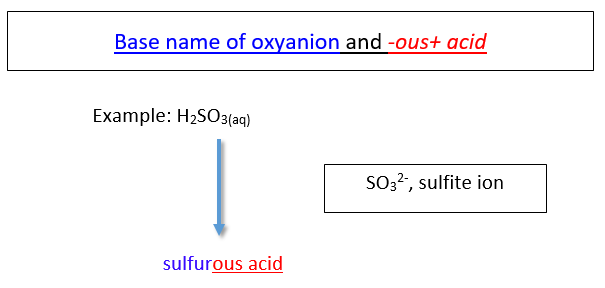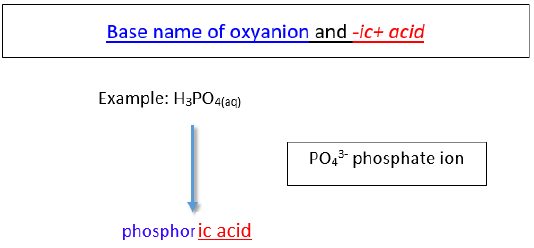Skip to main content

# 5.9: Naming Acids

A spot test for gold has been in use for decades. The sample is first treated with nitric acid. Other metals may react or dissolve in this acid, but gold will not. Then the sample is added to a mixture of nitric acid and hydrochloric acid. Gold will only dissolve in this mixture. The term "acid test" arose from the California gold rush in the late 1840's when this combination was used to test for the presence of real gold. It has since come to mean, "tested and approved" in a number of fields.

## Acids

An acid can be defined in several ways. The most straightforward definition is that an acid is a molecular compound that contains one or more hydrogen atoms and produces hydrogen ions $$\left( \ce{H^+} \right)$$ when dissolved in water.Figure $$\PageIndex{1}$$: (A) Vinegar comes in a variety of types, but all contain acetic acid. (B) Citrus fruits like grapefruit contain citric and ascorbic acids.

This is a different type of compound than the others we have seen so far. Acids are molecular, which means that in their pure state they are individual molecules and do not adopt the extended three-dimensional structures of ionic compounds like $$\ce{NaCl}$$. However, when these molecules are dissolved in water, the chemical bond between the hydrogen atom and the rest of the molecule breaks, leaving a positively-charged hydrogen ion and an anion. This can be symbolized in a chemical equation:

$\ce{HCl} \rightarrow \ce{H^+} + \ce{Cl^-}$

Since acids produce $$\ce{H^+}$$, cations upon dissolving in water, the $$\ce{H}$$ of an acid is written first in the formula of an inorganic acid. The remainder of the acid (other than the $$\ce{H}$$) is the anion after the acid dissolves. Organic acids are also an important class of compounds, but will not be discussed here.

## Naming Acids

Since all acids contain hydrogen, the name of an acid is based on the anion that goes with it. These anions can either be monatomic or polyatomic.

### Naming Binary acids (in aqueous form)

A binary acid is an acid that consists of hydrogen and one other element. The most common binary acids contain a halogen. The acid name begins with the prefix hydro-. followed by the base name of the anion, followed by the suffix -ic.### Naming Oxyacids

An oxyacid is an acid that consists of hydrogen, oxygen, and a third element. The third element is usually a nonmetal.

a. Oxyanions with -ite ending.

The name of the acid is the root of the anion followed by the suffix -ous. There is no prefix.b. Oxyanions with -ate ending.

The name of the acid is the root of the anion followed by the suffix -ic. There is no prefix.Note

The base name for sulfur containing oxyacid is sulfur- instead of just sulf-. The same is true for a phosphorus containing oxyacid. The base name is phosphor- instead of simply phosph-.

## Writing Formulas for Acids

Like other compounds that we have studied, acids are electrically neutral. Therefore, the charge of the anion part of the formula must be exactly balanced out by the $$\ce{H^+}$$ ions. Another way to think about writing the correct formula is to utilize the crisscross method, shown below for sulfuric acid.Formula: H2SO4

Figure $$\PageIndex{2}$$: Crisscross approach to writing formula for sulfuric acid.

## Summary

• Acids are molecular compounds that release hydrogen ions.
• A binary acid consists of hydrogen and one other element.
• Oxyacids contain hydrogen, oxygen, and one other element.
• The name of the acid is based on the anion attached to the hydrogen.

## Contributors

• CK-12 Foundation by Sharon Bewick, Richard Parsons, Therese Forsythe, Shonna Robinson, and Jean Dupon.

• Henry Agnew (UC Davis)